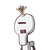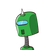# prove that opposite angles of a cyclic quadrilateral are supplementary​

prove that opposite angles of a cyclic quadrilateral are supplementary​

### 2 thoughts on “prove that opposite angles of a cyclic quadrilateral are supplementary​”

1.Step-by-step explanation:

The opposite angles in a cyclic quadrilateral are supplementary. i.e., the sum of the opposite angles is equal to 180˚.

Consider the diagram below.

If a, b, c, and d are the inscribed quadrilateral’s internal angles, then

a + b = 180˚ and c + d = 180˚.

Let’s prove that;

a + b = 180˚.

Join the vertices of the quadrilateral to the center of the circle.

Recall the inscribed angle theorem (the central angle = 2 x inscribed angle).

∠COD = 2∠CBD

∠COD = 2b

Similarly, by intercepted arc theorem,

∠COD = 2a

∠COD + reflex ∠COD = 360o

2a + 2b = 360o

2(a + b) =360o

By dividing both sides by 2, we get

a + b = 180o.

Hence proved!

2.[tex]\huge\bold{\mathtt{\red{A{\pink{N{\green{S{\blue{W{\purple{E{\orange{R}}}}}}}}}}}}}[/tex]